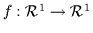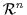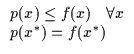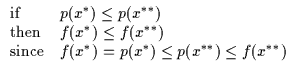Next: Quadratic Bounds Up: Optimization - General Bound Previous: Optimization - General Bound

# Bounding for Optimization

An important tool in optimization is bounding . Bounding can be used to compute upper or lower bounds on functions, approximation errors, etc. to tackle intractable maximization or minimization problems. The principles of bounding are central in variational methods which utilize bounds as approximation and optimization tools  . Of particular relevance are applications in statistics and graphical model learning as discussed by Rustagi  and Jordan . An interesting application is shown by Jaakkola  where bounds on Bayesian Networks are introduced to reduce intractable computations in a graphical model. We will discuss bounding techniques in a slightly different way, emphasizing quadratic bounds as opposed to the dual bounds typical of variational techniques and we occasionally refer back to some ideas used by classical variational methods in the literature.

Let us consider the usefulness of a bound for arbitrary function optimization. 6.1 Take a function, f, which maps a scalar, x, to a scalar, i.e.. For bounding purposes, the function's domain need not be restricted to this space and can be directly extended toand some non-Euclidean spaces as well. Now consider consider p which we shall call our contacting lower bound of f because of the properties in Equation 6.1.(6.1)

It can be shown that increasing p will also increase f as in Equation 6.2. This is a trivial proof however it guarantees that iteratively optimizing a function's bound is a useful alternative to directly optimizing the function. In the following, we can see that if we are currently operating at a point x* on function f, maximizing the bound p underneath f will make us move to another locus, x** where f is increased.(6.2)Next: Quadratic Bounds Up: Optimization - General Bound Previous: Optimization - General Bound
Tony Jebara
1999-09-15